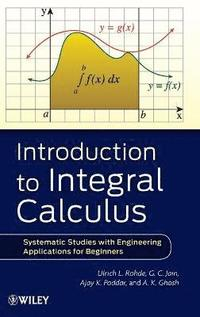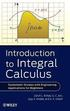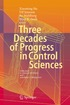Fler böcker inom
Format
Inbunden (Hardback)
Språk
Engelska
Antal sidor
432
Utgivningsdatum
2011-12-27
Upplaga
1
Förlag
John Wiley & Sons Inc
Illustrationer
Illustrations
Dimensioner
228 x 158 x 31 mm
Vikt
748 g
Antal komponenter
1
ISBN
9781118117767

### Du kanske gillar# Introduction to Integral Calculus

Systematic Studies with Engineering Applications for Beginners

Inbunden Engelska, 2011-12-27
1299
Skickas inom 5-8 vardagar.
Fri frakt inom Sverige för privatpersoner.
Finns även som
1729
1729
An accessible introduction to the fundamentals of calculus needed to solve current problems in engineering and the physical sciences I ntegration is an important function of calculus, and Introduction to Integral Calculus combines fundamental concepts with scientific problems to develop intuition and skills for solving mathematical problems related to engineering and the physical sciences. The authors provide a solid introduction to integral calculus and feature applications of integration, solutions of differential equations, and evaluation methods. With logical organization coupled with clear, simple explanations, the authors reinforce new concepts to progressively build skills and knowledge, and numerous real-world examples as well as intriguing applications help readers to better understand the connections between the theory of calculus and practical problem solving. The first six chapters address the prerequisites needed to understand the principles of integral calculus and explore such topics as anti-derivatives, methods of converting integrals into standard form, and the concept of area. Next, the authors review numerous methods and applications of integral calculus, including: * Mastering and applying the first and second fundamental theorems of calculus to compute definite integrals * Defining the natural logarithmic function using calculus * Evaluating definite integrals * Calculating plane areas bounded by curves * Applying basic concepts of differential equations to solve ordinary differential equations With this book as their guide, readers quickly learn to solve a broad range of current problems throughout the physical sciences and engineering that can only be solved with calculus. Examples throughout provide practical guidance, and practice problems and exercises allow for further development and fine-tuning of various calculus skills. Introduction to Integral Calculus is an excellent book for upper-undergraduate calculus courses and is also an ideal reference for students and professionals who would like to gain a further understanding of the use of calculus to solve problems in a simplified manner.

## Passar bra ihop

1.2. +
3.De som köpt den här boken har ofta också köpt Three Decades of Progress in Control Sciences av Xiaoming Hu, Ulf Jonsson, Bo Wahlberg, Bijoy Kumar Ghosh (inbunden).

## Kundrecensioner

Har du läst boken? Sätt ditt betyg »

## Recensioner i media

Introduction to Integral Calculus is an excellent book for upper-undergraduate calculus courses and is also an ideal reference for students and professionals who would like to gain a further understanding of the use of calculus to solve problems in a simplified manner. (Zentralblatt MATH, 2012) Long on examples but often short of exercises, this work might best be used as a reference source. Summing Up: Recommended. Lower-and upper-division undergraduates. (Choice, 1 September 2012)

## Övrig information

ULRICH L. ROHDE, PhD, ScD, Dr-Ing, is Chairman of Synergy Microwave Corporation, President of Communications Consulting Corporation, and a Partner of Rohde & Schwarz. A Fellow of the IEEE, Professor Rohde holds several patents and has published more than 200 scientific papers. G. C. JAIN, B.Sc., is a retired scientist from the Defense Research and Development Organization in India. AJAY K. PODDAR, PhD, is Chief Scientist at Synergy Microwave Corporation. A Senior Member of the IEEE, Dr. Poddar holds several dozen patents and has published more than 180 scientific papers. A. K. GHOSH, PhD, is Professor in the Department of Aerospace Engineering at the IIT Kanpur, India. He has published more than 120 scientific papers.

## Innehållsförteckning

FOREWORD ix PREFACE xiii BIOGRAPHIES xxi INTRODUCTION xxiii ACKNOWLEDGMENT xxv 1 Antiderivative(s) [or Indefinite Integral(s)] 1 1.1 Introduction 1 1.2 Useful Symbols, Terms, and Phrases Frequently Needed 6 1.3 Table(s) of Derivatives and their corresponding Integrals 7 1.4 Integration of Certain Combinations of Functions 10 1.5 Comparison Between the Operations of Differentiation and Integration 15 2 Integration Using Trigonometric Identities 17 2.1 Introduction 17 2.2 Some Important Integrals Involving sin x and cos x 34 2.3 Integrals of the Form ? (d/( a sin + b cos x)), where a, b r 37 3a Integration by Substitution: Change of Variable of Integration 43 3b Further Integration by Substitution: Additional Standard Integrals 67 4a Integration by Parts 97 4b Further Integration by Parts: Where the Given Integral Reappears on Right-Hand Side 117 5 Preparation for the Definite Integral: The Concept of Area 139 5.1 Introduction 139 5.2 Preparation for the Definite Integral 140 5.3 The Definite Integral as an Area 143 5.4 Definition of Area in Terms of the Definite Integral 151 5.5 Riemann Sums and the Analytical Definition of the Definite Integral 151 6a The Fundamental Theorems of Calculus 165 6b The Integral Function D x 1 1 t dt, (x > 0) Identified as ln x or loge x 183 7a Methods for Evaluating Definite Integrals 197 7b Some Important Properties of Definite Integrals 213 8a Applying the Definite Integral to Compute the Area of a Plane Figure 249 8b To Find Length(s) of Arc(s) of Curve(s), the Volume(s) of Solid(s) of Revolution, and the Area(s) of Surface(s) of Solid(s) of Revolution 295 9a Differential Equations: Related Concepts and Terminology 321 9a.4 Definition: Integral Curve 332 9b Methods of Solving Ordinary Differential Equations of the First Order and of the First Degree 361 INDEX 399